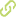# A cross-disciplinary introduction to quantum annealing-based algorithms Academic Article in Scopus•
• Overview
•
• Identity
•
• Additional Document Info
•
• View All
•

### abstract

• © 2018 Informa UK Limited, trading as Taylor & Francis Group. A central goal in quantum computing is the development of quantum hardware and quantum algorithms in order to analyse challenging scientific and engineering problems. Research in quantum computation involves contributions from both physics and computer science; hence this article presents a concise introduction to basic concepts from both fields that are used in annealing-based quantum computation, an alternative to the more familiar quantum gate model. We introduce some concepts from computer science required to define difficult computational problems and to realise the potential relevance of quantum algorithms to find novel solutions to those problems. We introduce the structure of quantum annealing-based algorithms as well as two examples of this kind of algorithms for solving instances of the max-SAT and Minimum Multicut problems. An overview of the quantum annealing systems manufactured by D-Wave Systems is also presented.
• © 2018 Informa UK Limited, trading as Taylor & Francis Group.A central goal in quantum computing is the development of quantum hardware and quantum algorithms in order to analyse challenging scientific and engineering problems. Research in quantum computation involves contributions from both physics and computer science; hence this article presents a concise introduction to basic concepts from both fields that are used in annealing-based quantum computation, an alternative to the more familiar quantum gate model. We introduce some concepts from computer science required to define difficult computational problems and to realise the potential relevance of quantum algorithms to find novel solutions to those problems. We introduce the structure of quantum annealing-based algorithms as well as two examples of this kind of algorithms for solving instances of the max-SAT and Minimum Multicut problems. An overview of the quantum annealing systems manufactured by D-Wave Systems is also presented.

### publication date

• April 3, 2018
• April 3, 2018